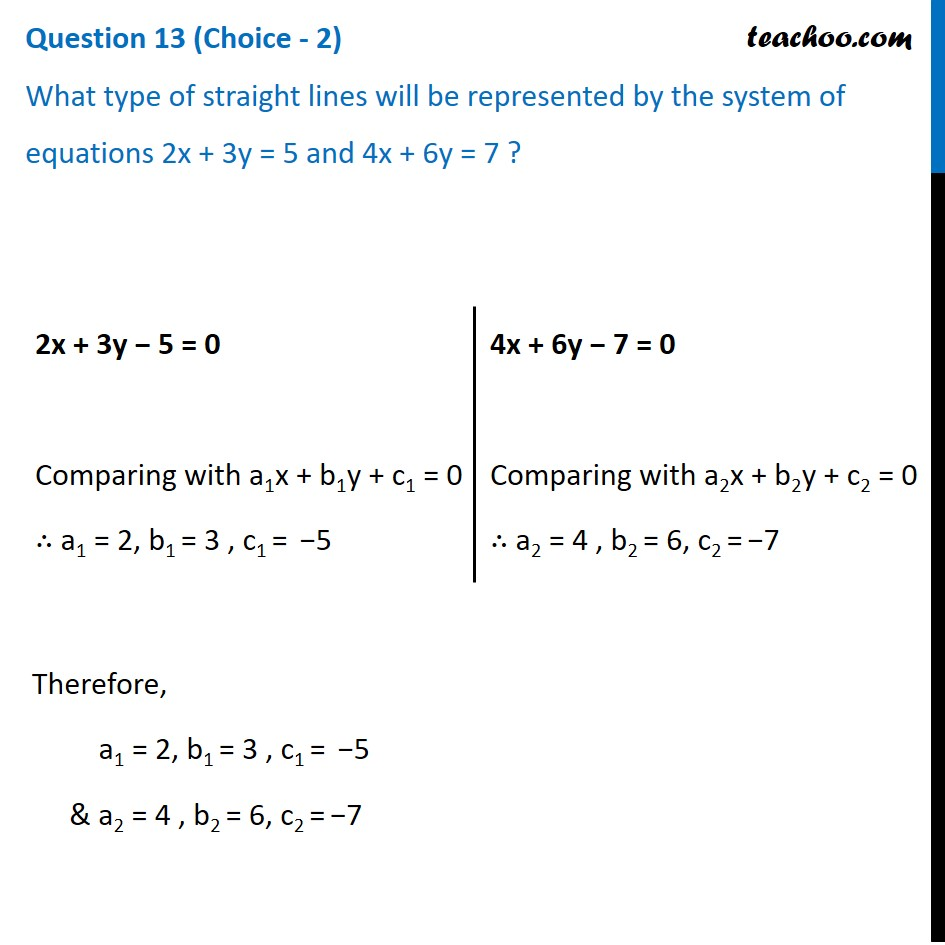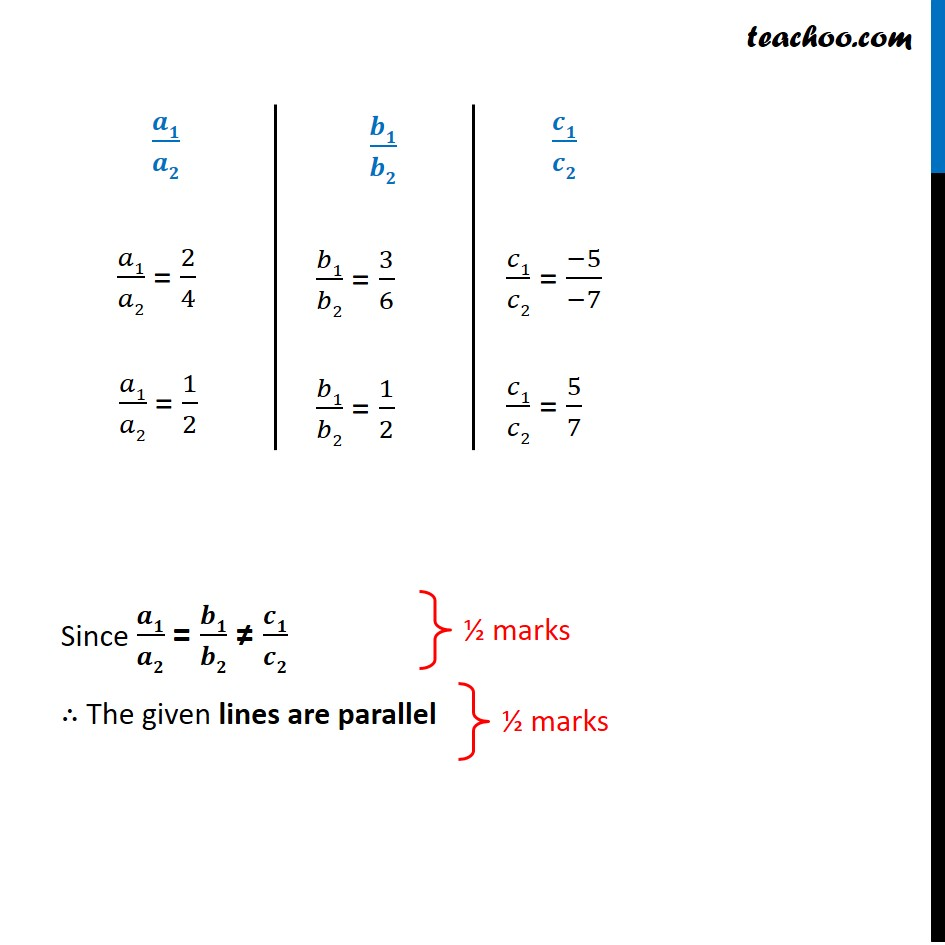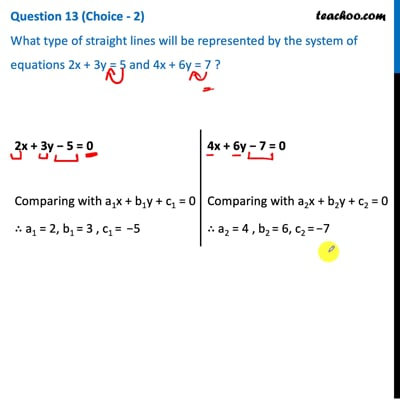CBSE Class 10 Sample Paper for 2021 Boards - Maths Basic

Class 10
Solutions of Sample Papers for Class 10 Boards

## What type of straight lines will be represented by the system of equations 2x + 3y = 5 and 4x + 6y = 7 ?This video is only available for Teachoo black users

### Transcript

Question 13 (Choice - 2) What type of straight lines will be represented by the system of equations 2x + 3y = 5 and 4x + 6y = 7 ?2x + 3y − 5 = 0 Comparing with a1x + b1y + c1 = 0 ∴ a1 = 2, b1 = 3 , c1 = −5 4x + 6y − 7 = 0 Comparing with a2x + b2y + c2 = 0 ∴ a2 = 4 , b2 = 6, c2 = −7 Therefore, a1 = 2, b1 = 3 , c1 = −5 & a2 = 4 , b2 = 6, c2 = −7 𝒂𝟏/𝒂𝟐 𝑎1/𝑎2 = 2/4 𝑎1/𝑎2 = 1/2 𝒃𝟏/𝒃𝟐 𝑏1/𝑏2 = 3/6 𝑏1/𝑏2 = 1/2 𝒄𝟏/𝒄𝟐 𝑐1/𝑐2 = (−5)/(−7) 𝑐1/𝑐2 = 5/7 Since 𝒂𝟏/𝒂𝟐 = 𝒃𝟏/𝒃𝟐 ≠ 𝒄𝟏/𝒄𝟐 ∴ The given lines are parallel